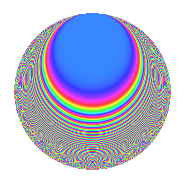# Properties

 Label 105.2.uLevel 105 Weight 2 Character orbit u Rep. character $$\chi_{105}(52,\cdot)$$ Character field $$\Q(\zeta_{12})$$ Dimension 32 Newform subspaces 1 Sturm bound 32 Trace bound 0

# Related objects

## Defining parameters

 Level: $$N$$ = $$105 = 3 \cdot 5 \cdot 7$$ Weight: $$k$$ = $$2$$ Character orbit: $$[\chi]$$ = 105.u (of order $$12$$ and degree $$4$$) Character conductor: $$\operatorname{cond}(\chi)$$ = $$35$$ Character field: $$\Q(\zeta_{12})$$ Newform subspaces: $$1$$ Sturm bound: $$32$$ Trace bound: $$0$$

## Dimensions

The following table gives the dimensions of various subspaces of $$M_{2}(105, [\chi])$$.

Total New Old
Modular forms 80 32 48
Cusp forms 48 32 16
Eisenstein series 32 0 32

## Trace form

 $$32q - 12q^{5} + 8q^{7} - 24q^{8} + O(q^{10})$$ $$32q - 12q^{5} + 8q^{7} - 24q^{8} - 12q^{10} - 8q^{11} - 8q^{15} - 8q^{21} - 8q^{22} - 8q^{23} + 12q^{25} + 24q^{26} - 24q^{28} + 8q^{30} + 24q^{31} + 24q^{32} - 36q^{33} + 44q^{35} - 32q^{36} + 4q^{37} + 12q^{38} + 12q^{40} + 16q^{42} + 40q^{43} - 40q^{46} - 60q^{47} + 72q^{50} - 8q^{51} - 108q^{52} - 24q^{53} - 48q^{56} + 16q^{57} + 4q^{58} + 20q^{60} - 24q^{61} + 4q^{63} - 4q^{65} + 72q^{66} + 8q^{67} + 132q^{68} + 4q^{70} - 16q^{71} + 12q^{72} + 36q^{73} + 48q^{75} + 60q^{77} + 80q^{78} - 12q^{80} + 16q^{81} + 12q^{82} - 72q^{85} - 16q^{86} - 24q^{87} - 32q^{88} - 24q^{91} - 56q^{92} - 24q^{93} - 12q^{95} - 72q^{98} + O(q^{100})$$

## Decomposition of $$S_{2}^{\mathrm{new}}(105, [\chi])$$ into newform subspaces

Label Dim. $$A$$ Field CM Traces $q$-expansion
$$a_2$$ $$a_3$$ $$a_5$$ $$a_7$$
105.2.u.a $$32$$ $$0.838$$ None $$0$$ $$0$$ $$-12$$ $$8$$

## Decomposition of $$S_{2}^{\mathrm{old}}(105, [\chi])$$ into lower level spaces

$$S_{2}^{\mathrm{old}}(105, [\chi]) \cong$$ $$S_{2}^{\mathrm{new}}(35, [\chi])$$$$^{\oplus 2}$$

## Hecke Characteristic Polynomials

There are no characteristic polynomials of Hecke operators in the database